# Blake et al. (2016) re-analysis

Blake, K. R., Dixson, B. J. W., O’Dean, S. M., & Denson, T. F. (2016). Standardized protocols for characterizing women’s fertility: A data-driven approach. Hormones and Behavior, 81, 74–83. doi:[10.1016/j.yhbeh.2016.03.004](https://dx.doi.org/10.1016/j.yhbeh.2016.03.004)

library(knitr)
opts_chunk$set(tidy = FALSE,cache = F, warning = F, message = F, render = formr::pander_handler) source("0_helpers.R") library(haven) conc = read_sav("blake_data/BDOD Conception probability data v2.sav") main = read_sav("blake_data/BDOD main data v3.sav") days6 = read_sav("blake_data/BDOD Six day window data v4.sav") ## Reproduce correlation for reported backward In paper: actual - FC: .11 actual - reported avg length BC: .35 actual - last length BC: .24 actual - last+avg length BC: .28 actual - avg all: .30 can only reproduce rank order, but they mentioned using some corrected correlation (because of groups) cor.test(conc$FCpred, conc$FCCP) Pearson’s product-moment correlation: conc$FCpred and conc$FCCP Test statistic df P value Alternative hypothesis 3.95 1440 0.00008179 * * * two.sided cor.test(conc$BCPred, conc$BCRepCP) Pearson’s product-moment correlation: conc$BCPred and conc$BCRepCP Test statistic df P value Alternative hypothesis 10.96 1142 1.19e-26 * * * two.sided cor.test(conc$BCPred, conc$BCPriorCP) Pearson’s product-moment correlation: conc$BCPred and conc$BCPriorCP Test statistic df P value Alternative hypothesis 7.753 1006 2.196e-14 * * * two.sided cor.test(conc$BCPred, conc$BCPriorRepAVCP) Pearson’s product-moment correlation: conc$BCPred and conc$BCPriorRepAVCP Test statistic df P value Alternative hypothesis 7.817 1016 1.349e-14 * * * two.sided cor.test(conc$BCPred, conc$BCAllMonCP) Pearson’s product-moment correlation: conc$BCPred and conc$BCAllMonCP Test statistic df P value Alternative hypothesis 8.18 1016 8.414e-16 * * * two.sided lmer(FCCP ~ FCpred + (1|Subject), data = conc) ## Linear mixed model fit by REML ['merModLmerTest'] ## Formula: FCCP ~ FCpred + (1 | Subject) ## Data: conc ## REML criterion at convergence: -3330 ## Random effects: ## Groups Name Std.Dev. ## Subject (Intercept) 0.00202 ## Residual 0.07587 ## Number of obs: 1442, groups: Subject, 103 ## Fixed Effects: ## (Intercept) FCpred ## 0.0565 0.1046 lmer(BCPred ~ BCRepCP + (1|Subject), data = conc) ## Linear mixed model fit by REML ['merModLmerTest'] ## Formula: BCPred ~ BCRepCP + (1 | Subject) ## Data: conc ## REML criterion at convergence: -2736 ## Random effects: ## Groups Name Std.Dev. ## Subject (Intercept) 0.0000 ## Residual 0.0727 ## Number of obs: 1144, groups: Subject, 103 ## Fixed Effects: ## (Intercept) BCRepCP ## 0.0609 0.3128 ## Reproduce T1 Not quite the same corrs. main %>% filter(!is.na(FCDay)) %>% select(LengthRep, LengthPrior,LengthPriorRepAV, LengthallMonthsRepAV, LengthTestingCycle) %>% cor(use='na.or.complete') %>% round(2) LengthRep LengthPrior LengthPriorRepAV LengthallMonthsRepAV LengthTestingCycle LengthRep 1 0.52 0.82 0.78 0.46 LengthPrior 0.52 1 0.91 0.86 0.56 LengthPriorRepAV 0.82 0.91 1 0.96 0.6 LengthallMonthsRepAV 0.78 0.86 0.96 1 0.58 LengthTestingCycle 0.46 0.56 0.6 0.58 1 main %>% filter(!is.na(FCDay)) %>% select(LengthRep, LengthPrior,LengthPriorRepAV, LengthallMonthsRepAV, LengthTestingCycle, FCDay, BCActual) %>% cor(use='na.or.complete') %>% round(2) LengthRep LengthPrior LengthPriorRepAV LengthallMonthsRepAV LengthTestingCycle FCDay BCActual LengthRep 1 0.52 0.82 0.78 0.46 0.48 0.12 LengthPrior 0.52 1 0.91 0.86 0.56 0.6 0.13 LengthPriorRepAV 0.82 0.91 1 0.96 0.6 0.62 0.15 LengthallMonthsRepAV 0.78 0.86 0.96 1 0.58 0.62 0.13 LengthTestingCycle 0.46 0.56 0.6 0.58 1 0.56 0.67 FCDay 0.48 0.6 0.62 0.62 0.56 1 -0.23 BCActual 0.12 0.13 0.15 0.13 0.67 -0.23 1 ## Descriptives of BC/FC ovulation day qplot(main$BCActual) + ggtitle("Which day was the surge day relative to next menstrual onset?")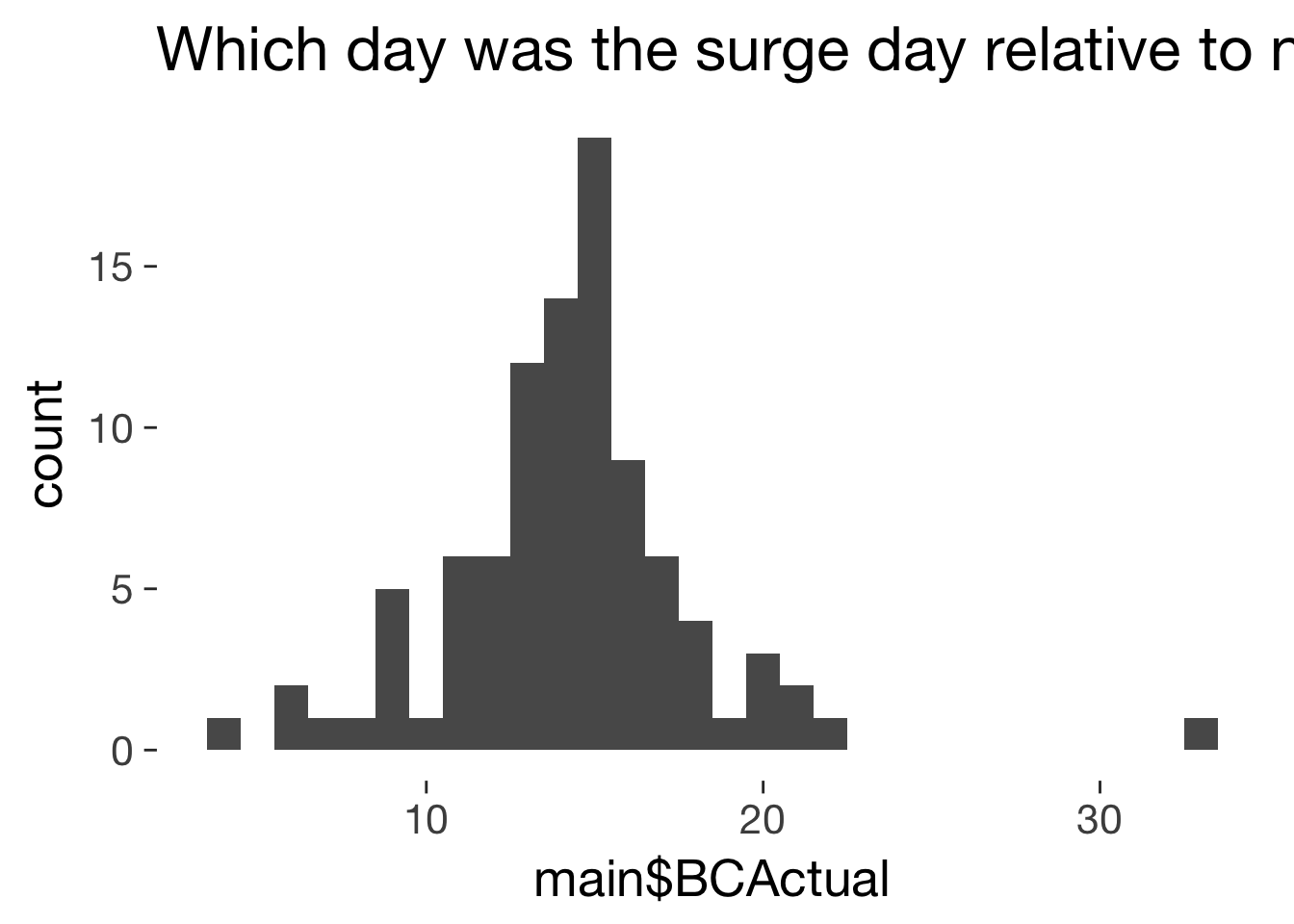mean(main$BCActual, na.rm = T) 14.26 sd(main$BCActual, na.rm = T)

3.813

mean(main$BCActual, na.rm = T, trim = 0.1) 14.21 mad(main$BCActual, na.rm = T)

2.965

qplot(main$FCDay)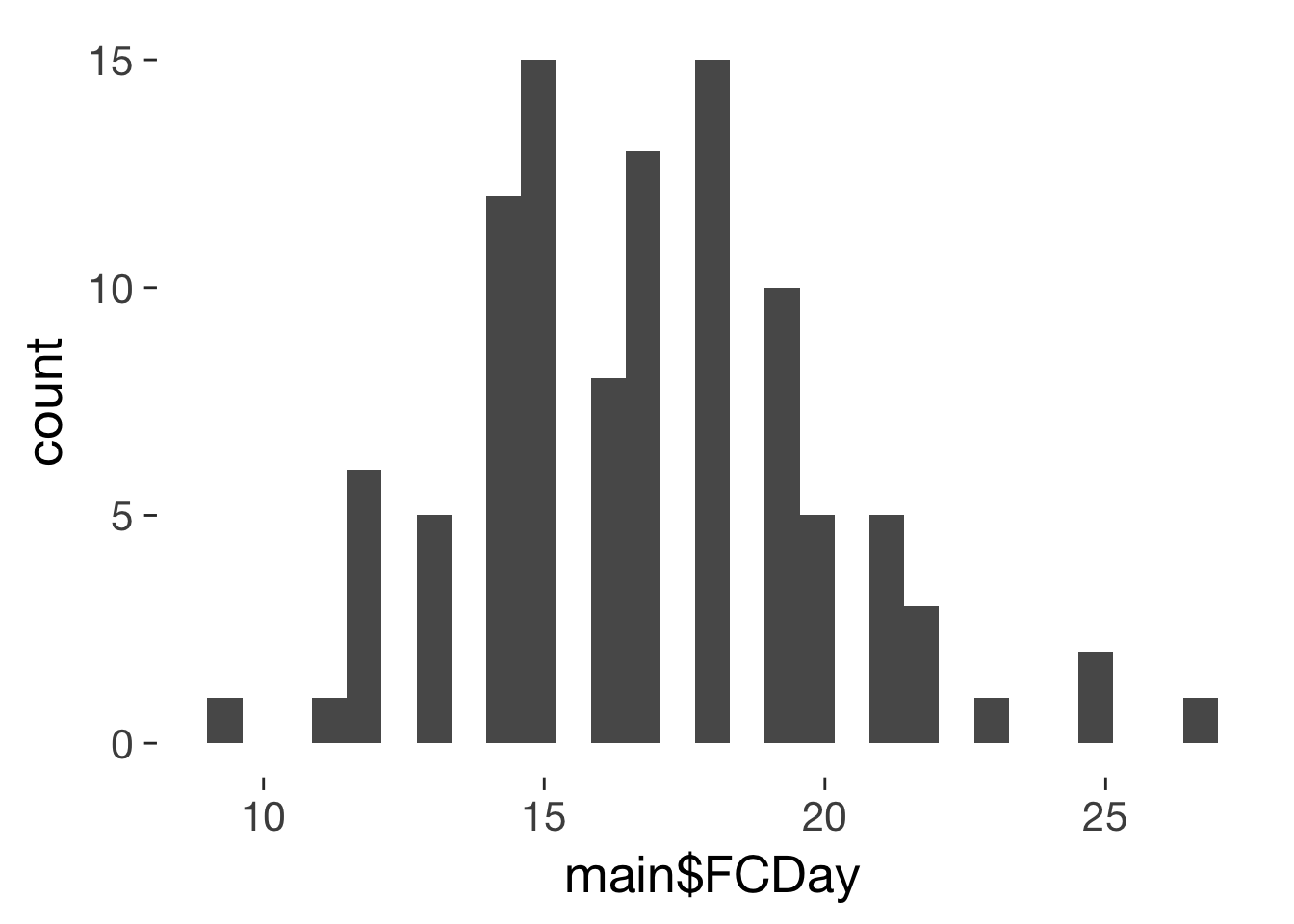mean(main$FCDay, na.rm = T)

16.8

sd(main$FCDay, na.rm = T) 3.163 mad(main$FCDay, na.rm = T)

2.965

## When does ovulation occur by cycle length?

main = main %>% filter(LengthTestingCycle<50)
xtabs(~ I(BCActual + FCDay - 1) == LengthTestingCycle, data = main)
TRUE
94
ggplot(main, aes(FCDay, BCActual)) + geom_jitter() + ggtitle("Pre-/post-surge, follicular v. luteal", subtitle = "The lengths of pre- and post-ovulation \nhalf are uncorrelated")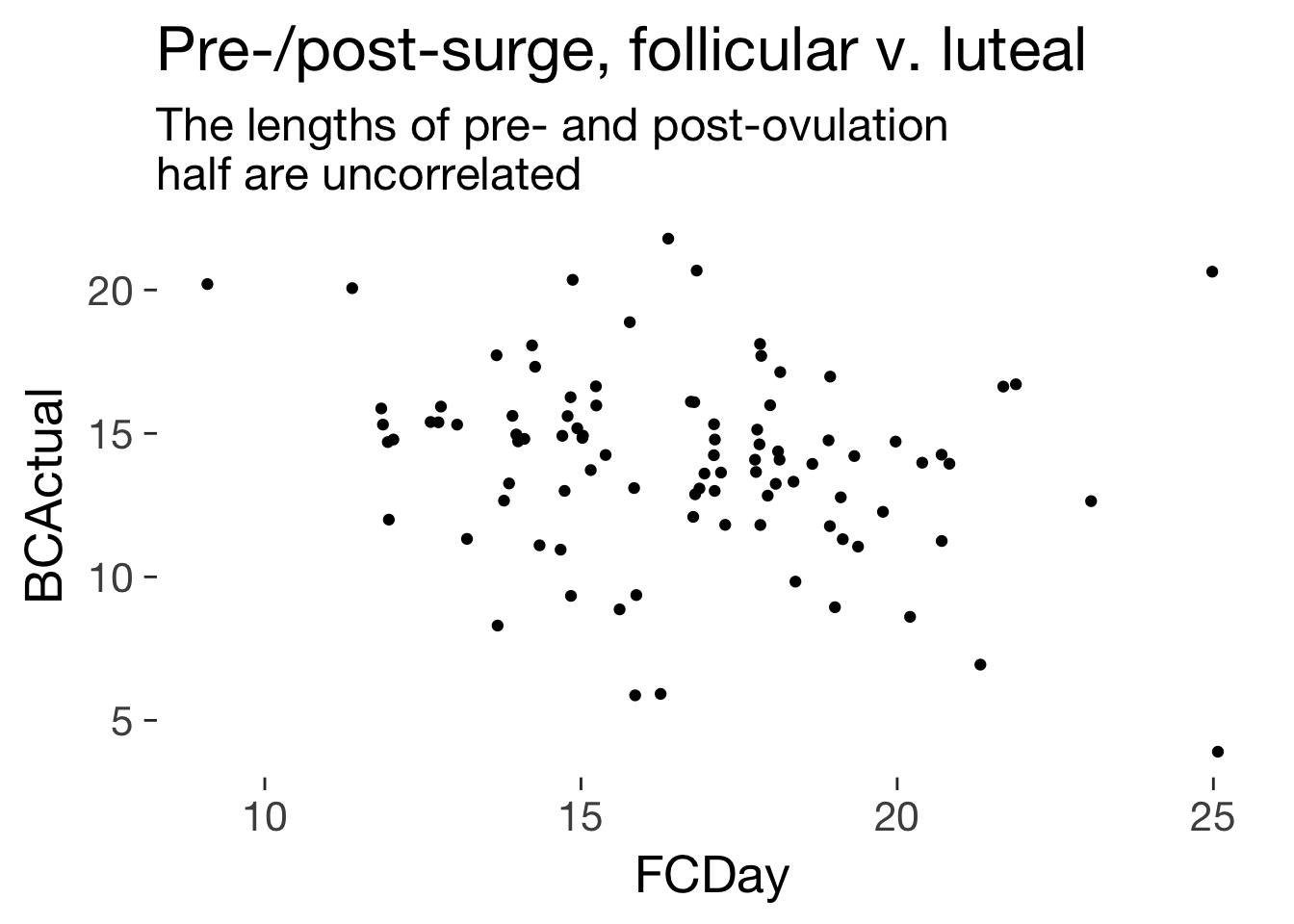ggplot(main, aes(LengthTestingCycle, BCActual)) + geom_jitter()+ ggtitle("Length of cycle and post-surge time", subtitle = "The longer the cycle, the earlier the ovulation") + geom_smooth(method = 'lm')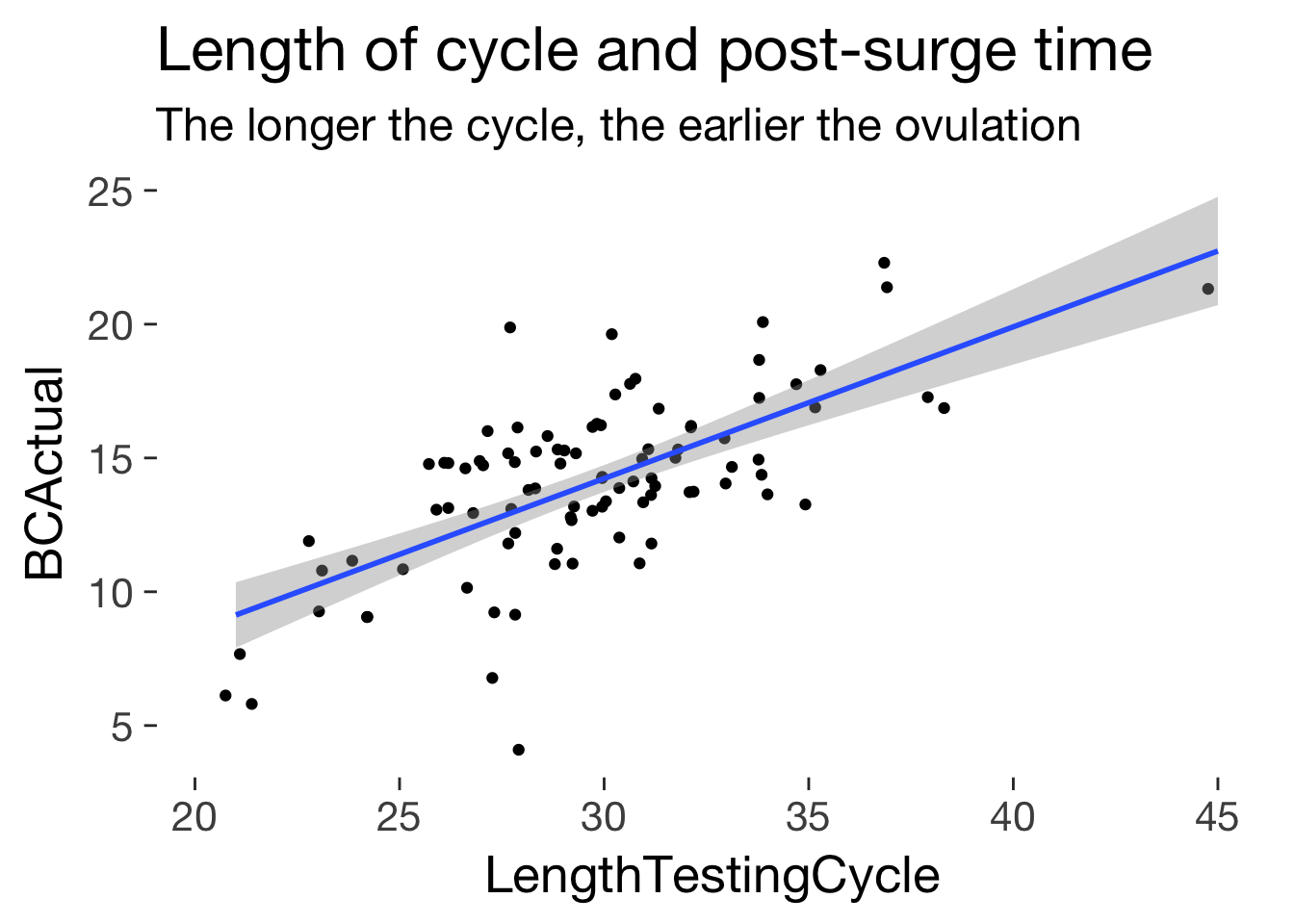ggplot(main, aes(LengthTestingCycle/1.7 - 3.5, BCActual)) + geom_jitter()+ ggtitle("Length of cycle and post-surge time", subtitle = "Ovulation tends to be in the cycle middle") + geom_smooth(method = 'lm') + geom_abline(slope = 1) + coord_fixed()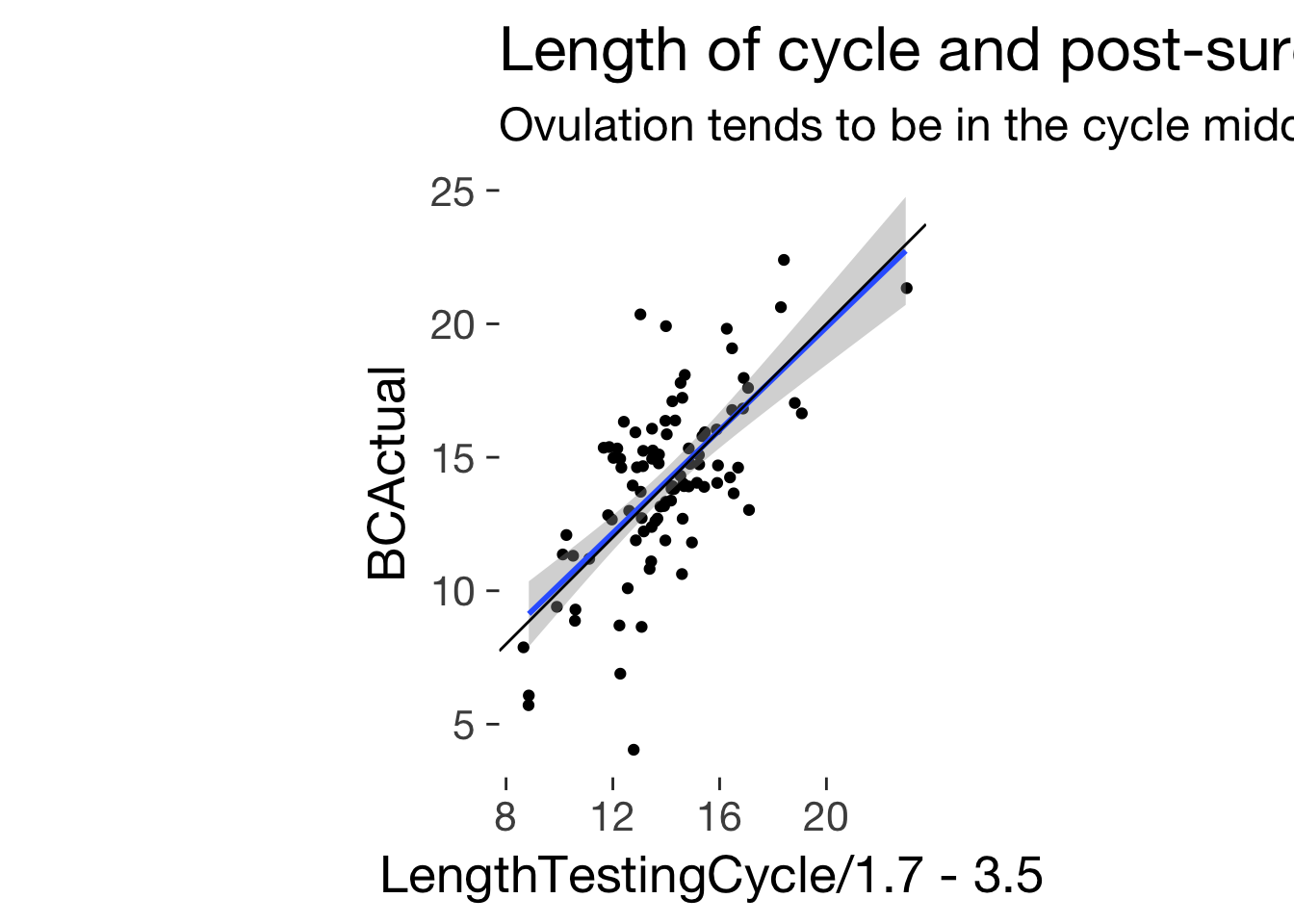lm(BCActual ~ LengthTestingCycle, data = main)
Fitting linear model: BCActual ~ LengthTestingCycle
Estimate Std. Error t value Pr(>|t|)
LengthTestingCycle 0.5668 0.06455 8.781 8.356e-14
(Intercept) -2.772 1.934 -1.434 0.155
cor.test(main$LengthTestingCycle, main$BCActual)
Pearson’s product-moment correlation: main$LengthTestingCycle and main$BCActual
Test statistic df P value Alternative hypothesis
8.781 92 8.356e-14 * * * two.sided
cor.test(main$LengthTestingCycle, main$FCDay)
Pearson’s product-moment correlation: main$LengthTestingCycle and main$FCDay
Test statistic df P value Alternative hypothesis
6.711 92 0.000000001556 * * * two.sided
lm(BCActual ~ I(LengthTestingCycle/2), data = main)
Fitting linear model: BCActual ~ I(LengthTestingCycle/2)
Estimate Std. Error t value Pr(>|t|)
I(LengthTestingCycle/2) 1.134 0.1291 8.781 8.356e-14
(Intercept) -2.772 1.934 -1.434 0.155
lm(BCActual ~ LengthTestingCycle, data = main %>% filter(LengthTestingCycle < 40))
Fitting linear model: BCActual ~ LengthTestingCycle
Estimate Std. Error t value Pr(>|t|)
LengthTestingCycle 0.5892 0.07078 8.324 8.123e-13
(Intercept) -3.415 2.106 -1.621 0.1084
lm(BCActual ~ I(LengthTestingCycle/2), data = main %>% filter(LengthTestingCycle < 40))
Fitting linear model: BCActual ~ I(LengthTestingCycle/2)
Estimate Std. Error t value Pr(>|t|)
I(LengthTestingCycle/2) 1.178 0.1416 8.324 8.123e-13
(Intercept) -3.415 2.106 -1.621 0.1084
lm(BCActual ~ I(LengthTestingCycle/2 - 3), data = main %>% filter(LengthTestingCycle < 40))
Fitting linear model: BCActual ~ I(LengthTestingCycle/2 - 3)
Estimate Std. Error t value Pr(>|t|)
I(LengthTestingCycle/2 - 3) 1.178 0.1416 8.324 8.123e-13
(Intercept) 0.1205 1.685 0.07152 0.9431
ggplot(main, aes(LengthTestingCycle/2, FCDay)) + geom_jitter()+ ggtitle("Length of cycle and pre-surge time", subtitle = "Ovulation tends to be in the cycle middle") + geom_smooth(method = 'lm')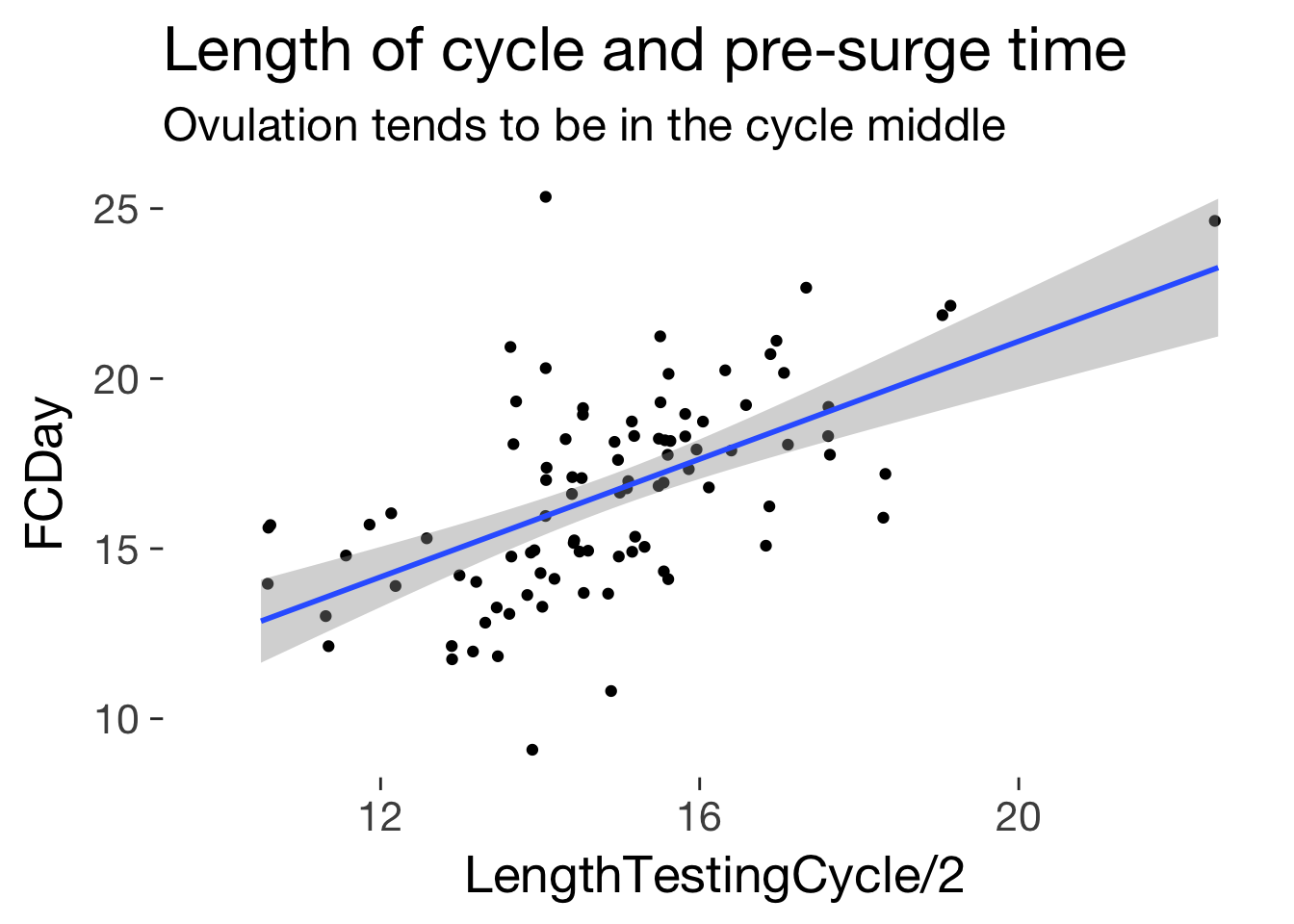lm(FCDay ~ I(LengthTestingCycle/2 + 5), data = main %>% filter(LengthTestingCycle < 40))
Fitting linear model: FCDay ~ I(LengthTestingCycle/2 + 5)
Estimate Std. Error t value Pr(>|t|)
I(LengthTestingCycle/2 + 5) 0.8216 0.1416 5.804 0.00000009353
(Intercept) 0.3069 2.81 0.1092 0.9133
ggplot(main, aes(LengthRep/2, FCDay)) + geom_jitter()+ ggtitle("Average reported cycle length and pre-surge time", subtitle = "Ovulation tends to be in the cycle middle") + geom_smooth(method = 'lm') + geom_abline(slope = 1) + coord_fixed()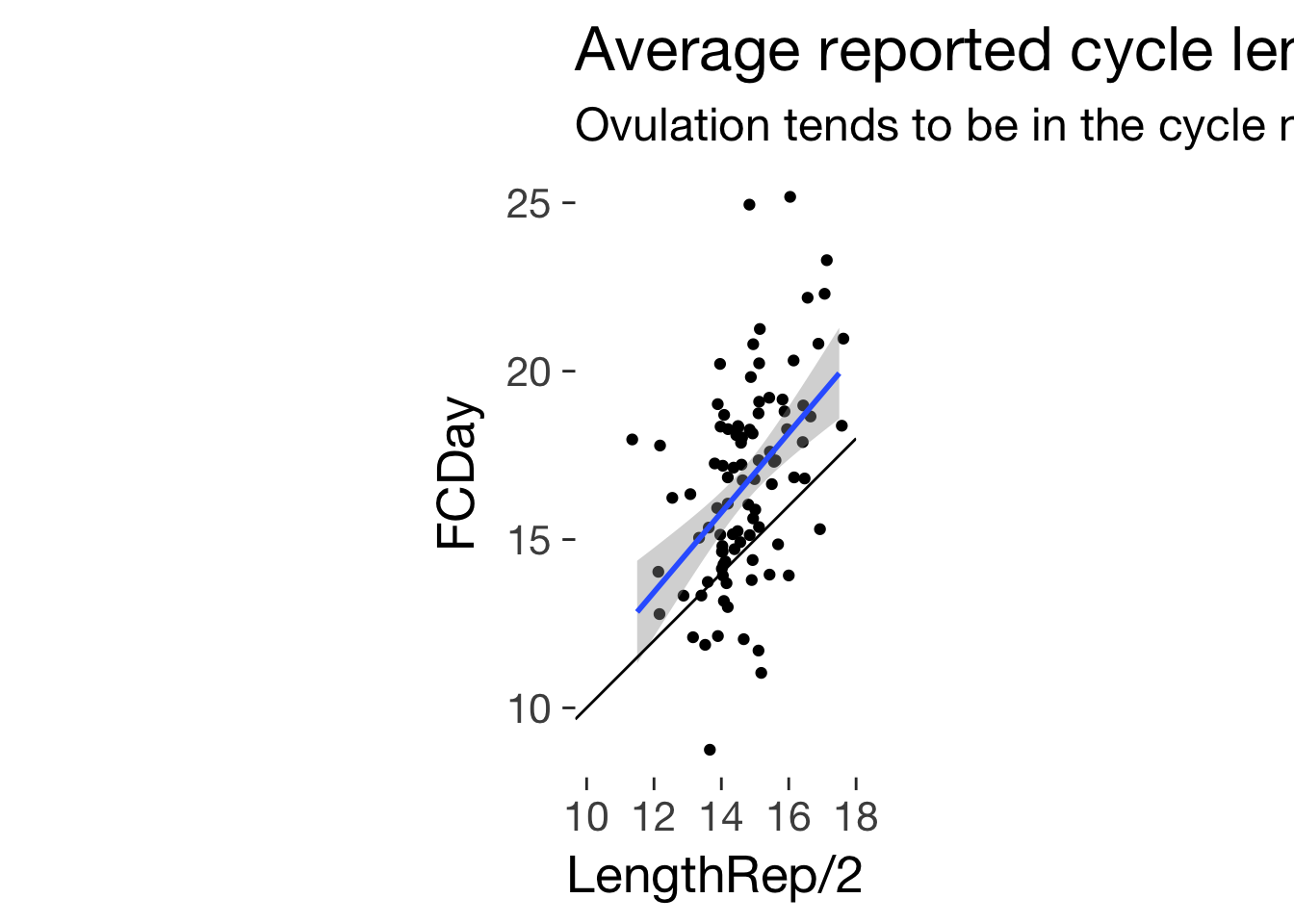lm(FCDay ~ I(LengthRep/2), data = main %>% filter(LengthTestingCycle < 40))
Fitting linear model: FCDay ~ I(LengthRep/2)
Estimate Std. Error t value Pr(>|t|)
I(LengthRep/2) 1.116 0.2175 5.13 0.000001624
(Intercept) 0.1557 3.206 0.04857 0.9614
cor.test(main$LengthRep, main$FCDay)
Pearson’s product-moment correlation: main$LengthRep and main$FCDay
Test statistic df P value Alternative hypothesis
5.285 92 0.0000008396 * * * two.sided
summary(lm(BCActual ~ LengthTestingCycle + LengthRep, data = main))
Estimate Std. Error t value Pr(>|t|)
LengthTestingCycle 0.664 0.07028 9.449 3.629e-15
LengthRep -0.3349 0.1139 -2.941 0.004146
(Intercept) 4.192 3.01 1.393 0.1671
Fitting linear model: BCActual ~ LengthTestingCycle + LengthRep
Observations Residual Std. Error $$R^2$$ Adjusted $$R^2$$
94 2.35 0.5032 0.4923

## Own approach

### get conception risk and and arrange by FCD

To do this we subtract 9 (as the 14 days are 8 days preceding ovulation, the day of ovulation, and 5 days after) and add 14 (to obtain days relative to menstrual onset). We then add days with 0 fertility for the remaining 40-14 days of the cycle.

conc_risks_rel_to_ovu = conc %>%
select(Index1, BCPred) %>% unique() %>%
mutate(Index1 = Index1 - 9)
qplot(Index1, BCPred, data = conc_risks_rel_to_ovu) + geom_vline(xintercept = 0)conc_risks_rel_to_ovu_interpolated = data.frame(Index1 = seq(-8, 5, 0.1)) %>%
left_join(conc_risks_rel_to_ovu)

library(mgcv)
m = gam(BCPred ~ s(Index1, k = 14), data = conc_risks_rel_to_ovu)
# plot(m)
conc_risks_rel_to_ovu_interpolated$BCPred2 = as.numeric(predict(m, newdata = conc_risks_rel_to_ovu_interpolated)) cor.test(conc_risks_rel_to_ovu_interpolated$BCPred,conc_risks_rel_to_ovu_interpolated$BCPred2) Pearson’s product-moment correlation: conc_risks_rel_to_ovu_interpolated$BCPred and conc_risks_rel_to_ovu_interpolated$BCPred2 Test statistic df P value Alternative hypothesis 84.72 12 4.882e-18 * * * two.sided normal_curve = function(x, sd, mean) { exp(- ( (x - mean)^2/(2 * sd^2) )) / sqrt( 2*sd^2 * pi ) } conc_risks_rel_to_ovu_interpolated$BCPred_normal = 1.09*normal_curve(conc_risks_rel_to_ovu_interpolated$Index1, sd = 2, mean = -1.3) conc_risks_rel_to_ovu_interpolated %>% gather(key,value,-Index1) %>% na.omit() %>% ggplot(aes(Index1, value, color = key)) + geom_line()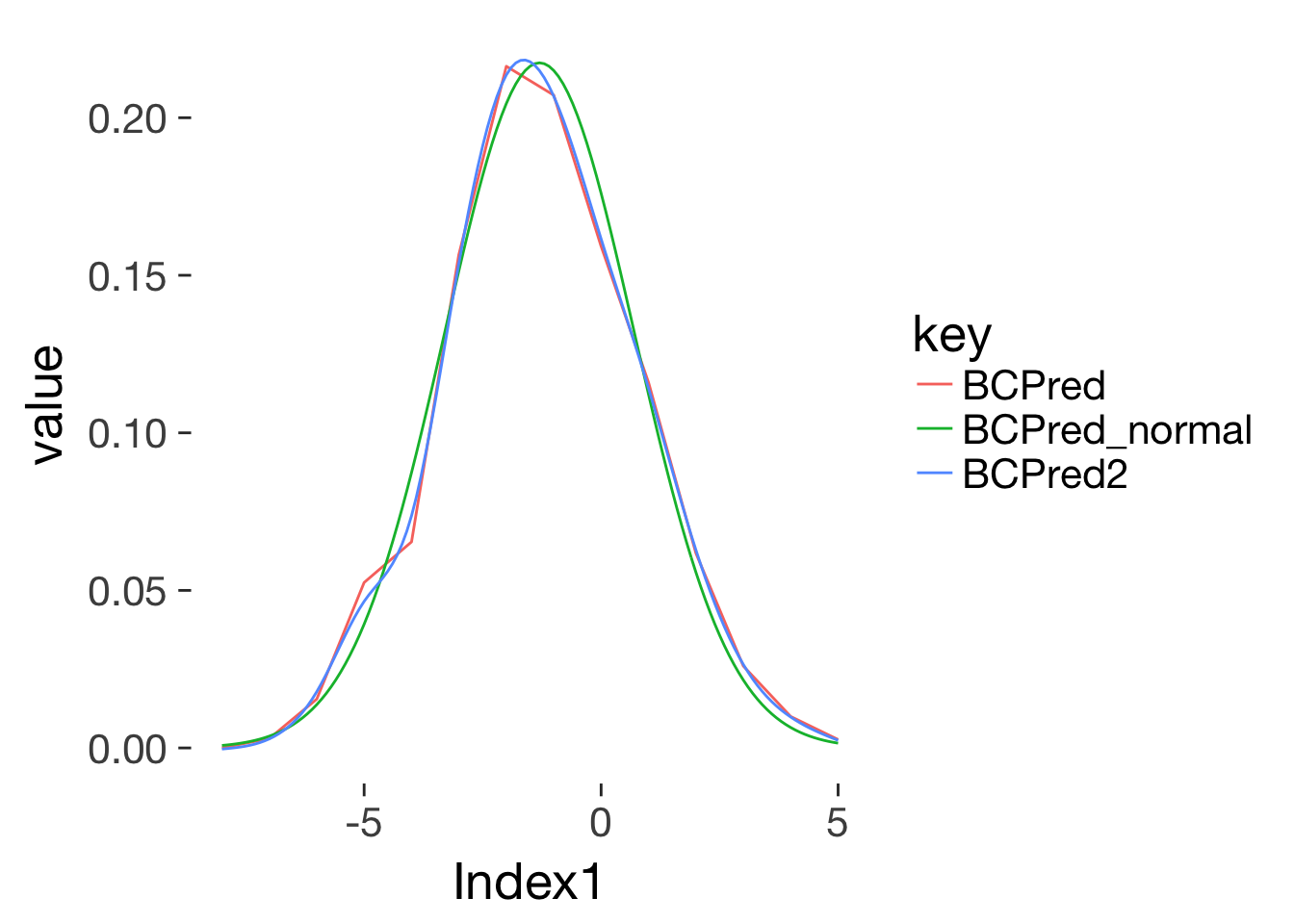### fertile window probabilities from gangestad ea. 2006 days = data.frame( RCD_for_merge = c(29:1, 30:41), FCD = c(1:41), prc_stirn_b = c(.01, .01, .02, .03, .05, .09, .16, .27, .38, .48, .56, .58, .55, .48, .38, .28, .20, .14, .10, .07, .06, .04, .03, .02, .01, .01, .01, .01, .01, rep(.01, times = 12)), # rep(.01, times = 70)), # gangestad uses .01 here, but I think such cases are better thrown than kept, since we might simply have missed a mens prc_wcx_b = c(.000, .000, .001, .002, .004, .009, .018, .032, .050, .069, .085, .094, .093, .085, .073, .059, .047, .036, .028, .021, .016, .013, .010, .008, .007, .006, .005, .005, .005, rep(.005, times = 12)) ) days$RCD = -1 * days$RCD_for_merge + 15 m = gam(prc_stirn_b ~ s(RCD, k = 14), data = days) # plot(m) days$prc_stirn_b2 = as.numeric(predict(m, newdata = days))
cor.test(days$prc_stirn_b,days$prc_stirn_b2)
Pearson’s product-moment correlation: days$prc_stirn_b and days$prc_stirn_b2
Test statistic df P value Alternative hypothesis
536.7 39 4.65e-77 * * * two.sided
days$prc_stirn_b = days$prc_stirn_b2

### expand the main dataset into long format

Essentially we’re trying to reproduce the conception risk dataset.

Best guesses as to what variables mean: - LengthTestingCycle: The length of the cycle in which women actually used the LH strips. - BCActual: The day the LH surge occurred, counted from the end of the cycle - FCDay: The day the LH surge occurred, counted from the beginning of the cycle - LengthRep: The average cycle length reported in the screening survey.

conc_risks_rel_to_ovu = conc_risks_rel_to_ovu_interpolated %>% select(Index1, BCPred2) %>% rename(BCPred = BCPred2)
long = main %>% select(Subject, Age, RelStat, LengthTestingCycle, FCDay, BCActual, LengthRep) %>%   filter(!is.na(LengthTestingCycle)) %>%  # can't use those without complete cycle
group_by(Subject, Age, RelStat, LengthTestingCycle, FCDay, BCActual, LengthRep) %>%
# group to retain after expanding
expand(FCD = full_seq(1:LengthTestingCycle, period = 1)) %>%
mutate(
day_of_ovu_surge = FCDay + 1, # ovulation occurs one day after LH surge
day_of_ovu_bc_est_m14 = LengthTestingCycle - 1 - 14, # counting 14 back from the next menstrual onset, minus 1 cause we're translating a length into days from 0
day_of_ovu_fc_est_m14 = LengthRep - 1 - 14, # counting 14 back from the next menstrual onset, minus 1 cause we're translating a length into days from 0
day_of_ovu_fc = 14,
day_of_ovu_bc_est_half = (LengthTestingCycle - 1)/2 + 3, # three days after the middle of the cycle
day_of_ovu_rep_half = (LengthRep - 1)/2 + 3, # three days after the middle of the cycle

FCD0 = FCD - 1, # if we want to subtract the day of ovu, need to start counting from 0
days_until_ovu_bc_half = FCD0 - day_of_ovu_bc_est_half ,
days_until_ovu_rep_half = FCD0 - day_of_ovu_rep_half,
days_until_ovu_bc_m14 = FCD0 - day_of_ovu_bc_est_m14,
days_until_ovu_fc_m14 = FCD0 - day_of_ovu_fc_est_m14,
days_until_ovu_fc = FCD0 - day_of_ovu_fc,
days_until_ovu_surge = FCD0 - day_of_ovu_surge,
days_until_ovu_bc_m14_squished = if_else(
LengthTestingCycle - FCD < 14,
29 - (LengthTestingCycle - FCD),
round((FCD/ (LengthTestingCycle - 14) ) * 15)) - 15
) %>%
left_join(conc_risks_rel_to_ovu %>% rename(conc_bc_m14 = BCPred), by = c("days_until_ovu_bc_m14" = "Index1")) %>%
left_join(conc_risks_rel_to_ovu %>% rename(conc_bc_m14_sq = BCPred), by = c("days_until_ovu_bc_m14_squished" = "Index1")) %>%
left_join(days %>% select(RCD, prc_stirn_b) %>% rename(fert_bc_m14_sq = prc_stirn_b), by = c("days_until_ovu_bc_m14_squished" = "RCD")) %>%
left_join(days %>% select(RCD, prc_stirn_b) %>% rename(fert_bc_m14 = prc_stirn_b), by = c("days_until_ovu_bc_m14" = "RCD")) %>%
left_join(conc_risks_rel_to_ovu %>% rename(conc_fc_m14 = BCPred), by = c("days_until_ovu_fc_m14" = "Index1")) %>%
left_join(days %>% select(RCD, prc_stirn_b) %>% rename(fert_fc_m14 = prc_stirn_b), by = c("days_until_ovu_fc_m14" = "RCD")) %>%
left_join(conc_risks_rel_to_ovu %>% rename(conc_ovu_fc = BCPred), by = c("days_until_ovu_fc" = "Index1")) %>%
left_join(conc_risks_rel_to_ovu %>% rename(conc_ovu_surge = BCPred), by = c("days_until_ovu_surge" = "Index1")) %>%
left_join(days %>% select(RCD, prc_stirn_b) %>% rename(fert_ovu_surge = prc_stirn_b), by = c("days_until_ovu_surge" = "RCD")) %>%
mutate(
conc_bc_m14 = if_else(is.na(conc_bc_m14), 0, conc_bc_m14),
conc_fc_m14 = if_else(is.na(conc_fc_m14), 0, conc_fc_m14),
conc_ovu_fc = if_else(is.na(conc_ovu_fc), 0, conc_ovu_fc),
conc_ovu_surge = if_else(is.na(conc_ovu_surge), 0, conc_ovu_surge)
) %>%
ungroup()

# View(long %>% select(Subject, FCD, RCD, ovu_day_fcd, ovu_day_rcd) %>% filter(ovu_day_rcd|ovu_day_fcd))
# ggplot(long %>% filter(Subject==2), aes(days_until_ovu_rep_half, conc_rep_half))+geom_point() + geom_vline(xintercept=0)
# ggplot(long %>% filter(Subject==2), aes(days_until_ovu_bc_m14, conc_bc_m14))+geom_point() + geom_vline(xintercept=0)
# ggplot(long %>% filter(Subject==2), aes(days_until_ovu_fc, conc_ovu_fc))+geom_point() + geom_vline(xintercept=0)
# ggplot(long %>% filter(Subject==2), aes(days_until_ovu_surge, conc_ovu_surge))+geom_point() + geom_vline(xintercept=0)

# ggplot(long %>% filter(Subject==2), aes(days_until_ovu_bc_half, days_until_ovu_surge))+geom_point() + geom_abline(slope = 1)
# ggplot(long %>% filter(Subject==2), aes(days_until_ovu_bc_m14, days_until_ovu_surge))+geom_point() + geom_abline(slope = 1)

### conception risk correlations

long %>% select(starts_with("conc_")) %>% cor(use='pairwise.complete.obs') %>% round(2)# %>% .[,5]
conc_bc_m14 conc_bc_m14_sq conc_fc_m14 conc_ovu_fc conc_ovu_surge
conc_bc_m14 1 0.94 0.54 0.49 0.36
conc_bc_m14_sq 0.94 1 0.4 0.36 0.24
conc_fc_m14 0.54 0.4 1 0.66 0.34
conc_ovu_fc 0.49 0.36 0.66 1 0.29
conc_ovu_surge 0.36 0.24 0.34 0.29 1

### fertile window probability correlations

The day of the next menstrual onset minus 14 (with “squishing” of fertile phase probabilities in the follicular phase) is the index we used in our manuscript fert_bc_m14_sq. fert_ovu_surge is the probability of being in the fertile window when using the day of ovulation estimated using the LH surge.

print(cor.test(long$fert_bc_m14_sq, long$fert_ovu_surge)) # does not properly treat the nested structure of the data
##
##  Pearson's product-moment correlation
##
## data:  long$fert_bc_m14_sq and long$fert_ovu_surge
## t = 37, df = 2800, p-value <2e-16
## alternative hypothesis: true correlation is not equal to 0
## 95 percent confidence interval:
##  0.5456 0.5959
## sample estimates:
##    cor
## 0.5713
long %>% group_by(Subject) %>%
filter(!is.na(fert_bc_m14_sq), !is.na(fert_ovu_surge)) %>%
summarise(corr = cor(fert_bc_m14_sq, fert_ovu_surge, use = 'pairwise.complete.obs')) %>%
ungroup() %>%
summarise(cor = mean(corr), cor_sd = sd(corr), n = n()) %>% # to get a sense of interindividual variation in the corr
pander::pander()
cor cor_sd n
0.5536 0.4188 94
long %>% select(starts_with("fert_")) %>% cor(use='pairwise.complete.obs') %>% round(2)# %>% .[,5]
fert_bc_m14_sq fert_bc_m14 fert_fc_m14 fert_ovu_surge
fert_bc_m14_sq 1 0.94 0.72 0.57
fert_bc_m14 0.94 1 0.66 0.56
fert_fc_m14 0.72 0.66 1 0.53
fert_ovu_surge 0.57 0.56 0.53 1

#### subset to cycles 25-33

long %>% filter(LengthTestingCycle %in% 25:33)  %>% select(starts_with("conc_")) %>% cor(use='na.or.complete') %>% round(2)
conc_bc_m14 conc_bc_m14_sq conc_fc_m14 conc_ovu_fc conc_ovu_surge
conc_bc_m14 1 0.98 0.55 0.59 0.22
conc_bc_m14_sq 0.98 1 0.56 0.65 0.22
conc_fc_m14 0.55 0.56 1 0.56 0.2
conc_ovu_fc 0.59 0.65 0.56 1 0.1
conc_ovu_surge 0.22 0.22 0.2 0.1 1

### mean fertility on day of ovulation

long %>% filter(days_until_ovu_surge %in% -2:0) %>%
ungroup() %>%
select(starts_with("conc")) %>%
summarise_each(funs(mean)) %>% pander()
conc_bc_m14 conc_bc_m14_sq conc_fc_m14 conc_ovu_fc conc_ovu_surge
0.07985 NA 0.07522 0.07029 0.194

### days until ovulation

long %>% select(starts_with("days_until_ovu")) %>% cor(use='na.or.complete') %>% round(2)
days_until_ovu_bc_half days_until_ovu_rep_half days_until_ovu_bc_m14 days_until_ovu_fc_m14 days_until_ovu_fc days_until_ovu_surge days_until_ovu_bc_m14_squished
days_until_ovu_bc_half 1 0.98 0.98 0.97 0.98 0.96 0.98
days_until_ovu_rep_half 0.98 1 0.92 0.99 0.99 0.96 0.95
days_until_ovu_bc_m14 0.98 0.92 1 0.92 0.9 0.94 0.98
days_until_ovu_fc_m14 0.97 0.99 0.92 1 0.96 0.95 0.94
days_until_ovu_fc 0.98 0.99 0.9 0.96 1 0.95 0.93
days_until_ovu_surge 0.96 0.96 0.94 0.95 0.95 1 0.94
days_until_ovu_bc_m14_squished 0.98 0.95 0.98 0.94 0.93 0.94 1
long %>% filter(!duplicated(Subject)) %>% select(starts_with("day_of_ovu"))  %>% ungroup() %>%
gather(key, value, -day_of_ovu_surge) %>%
group_by(key) %>%
do(cbind(mean_v =mean(.$value), describe(.$value - .\$day_of_ovu_surge))) %>%
select(key, mean_v, mean, sd, median, min, max) %>%
rename(mean_diff = mean, mean = mean_v) %>%
pander()
key mean mean_diff sd median min max
day_of_ovu_bc_est_half 17.24 -0.2872 2.447 -0.5 -9.5 6.5
day_of_ovu_bc_est_m14 14.49 -2.936 3.298 -3 -13 5
day_of_ovu_fc 14 -3.638 2.969 -4 -12 4
day_of_ovu_fc_est_m14 14.14 -3.223 2.783 -3 -11 3
day_of_ovu_rep_half 17.07 -0.4309 2.61 -0.5 -8.5 6
xsect = long %>% filter(!duplicated(Subject)) %>% ungroup()
qplot(day_of_ovu_surge - day_of_ovu_bc_est_m14, data=xsect, binwidth = 0.5)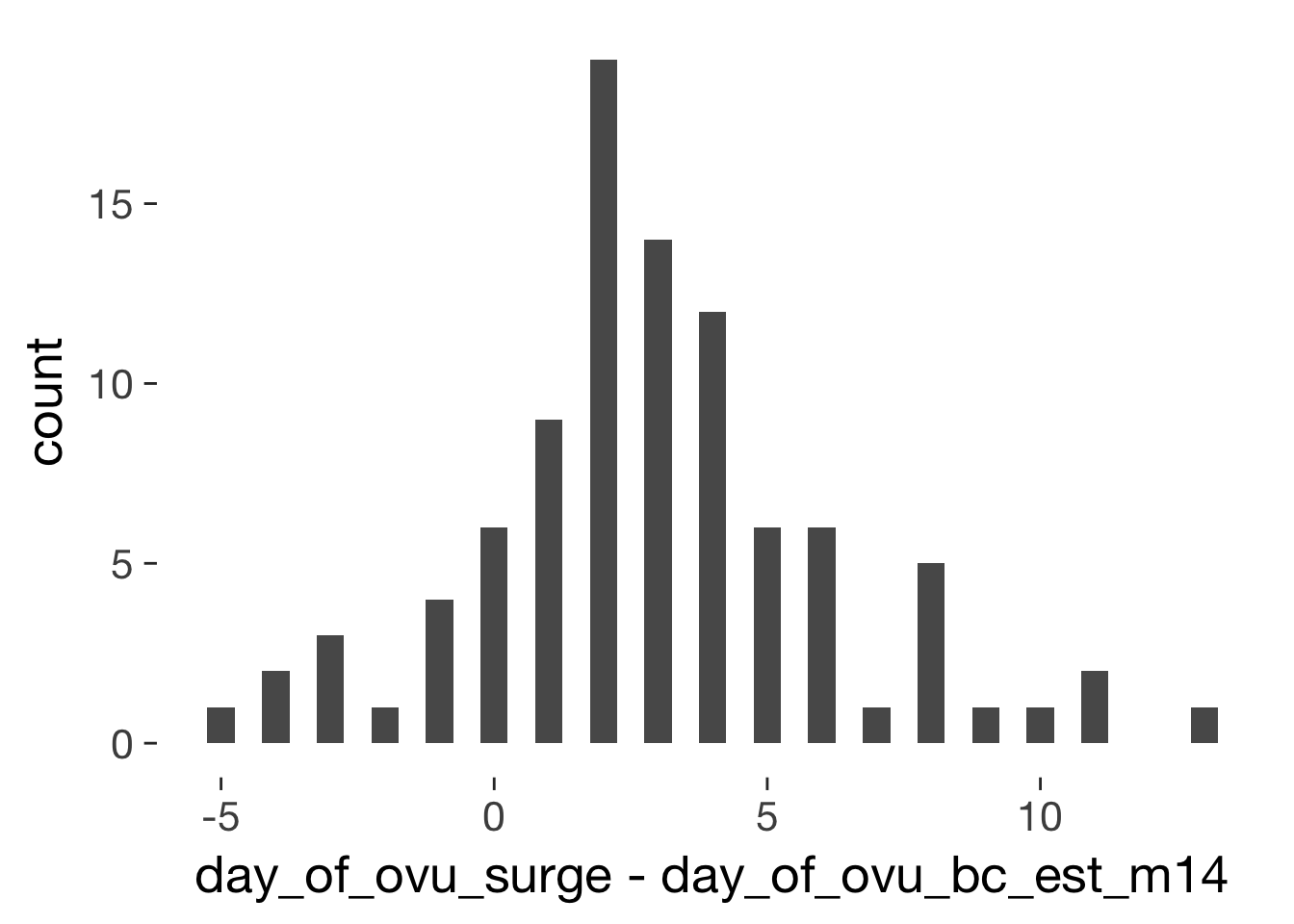qplot(day_of_ovu_surge - day_of_ovu_rep_half, data=xsect, binwidth = 0.5)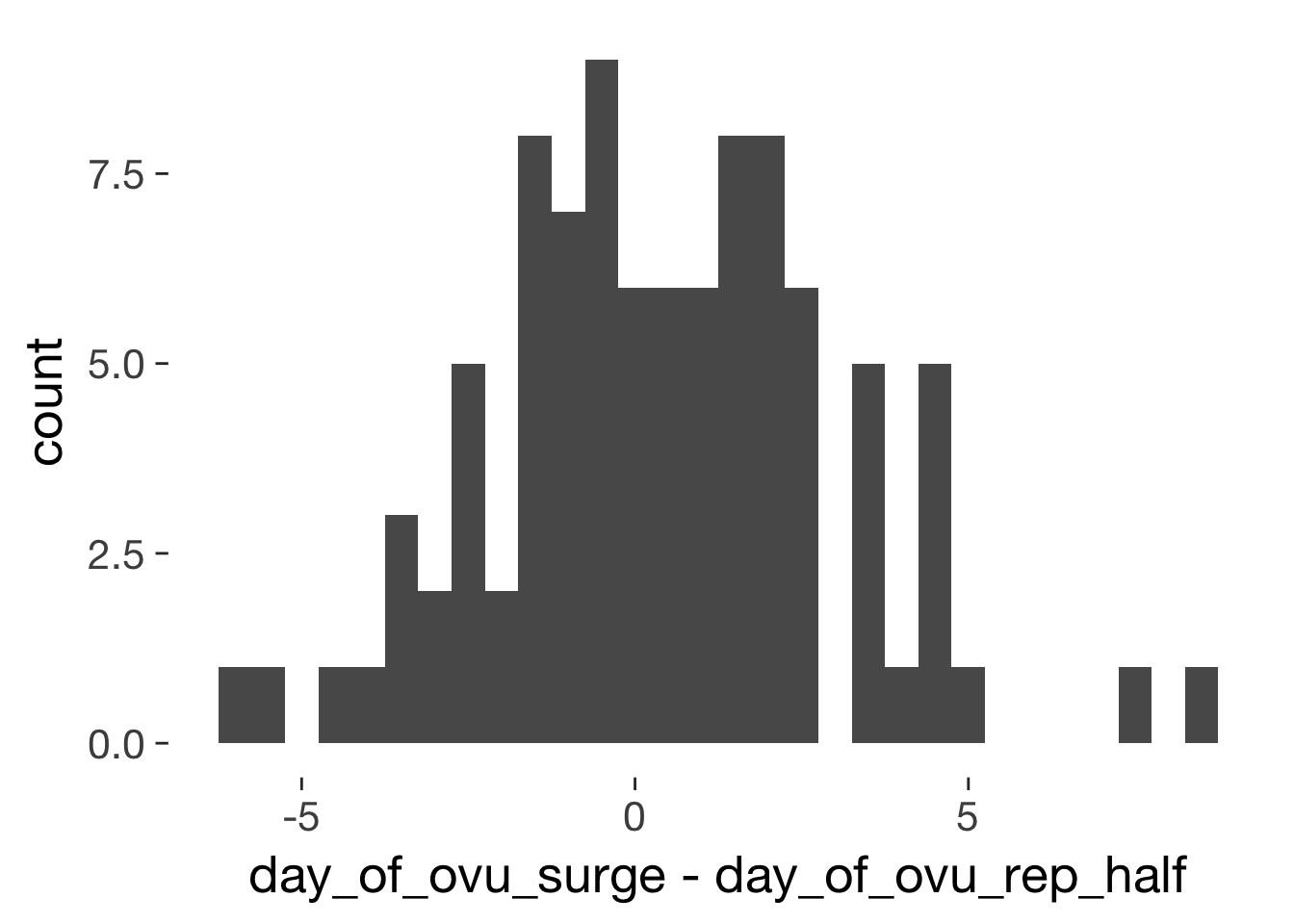qplot(day_of_ovu_surge - day_of_ovu_bc_est_half, data=xsect, binwidth = 0.5)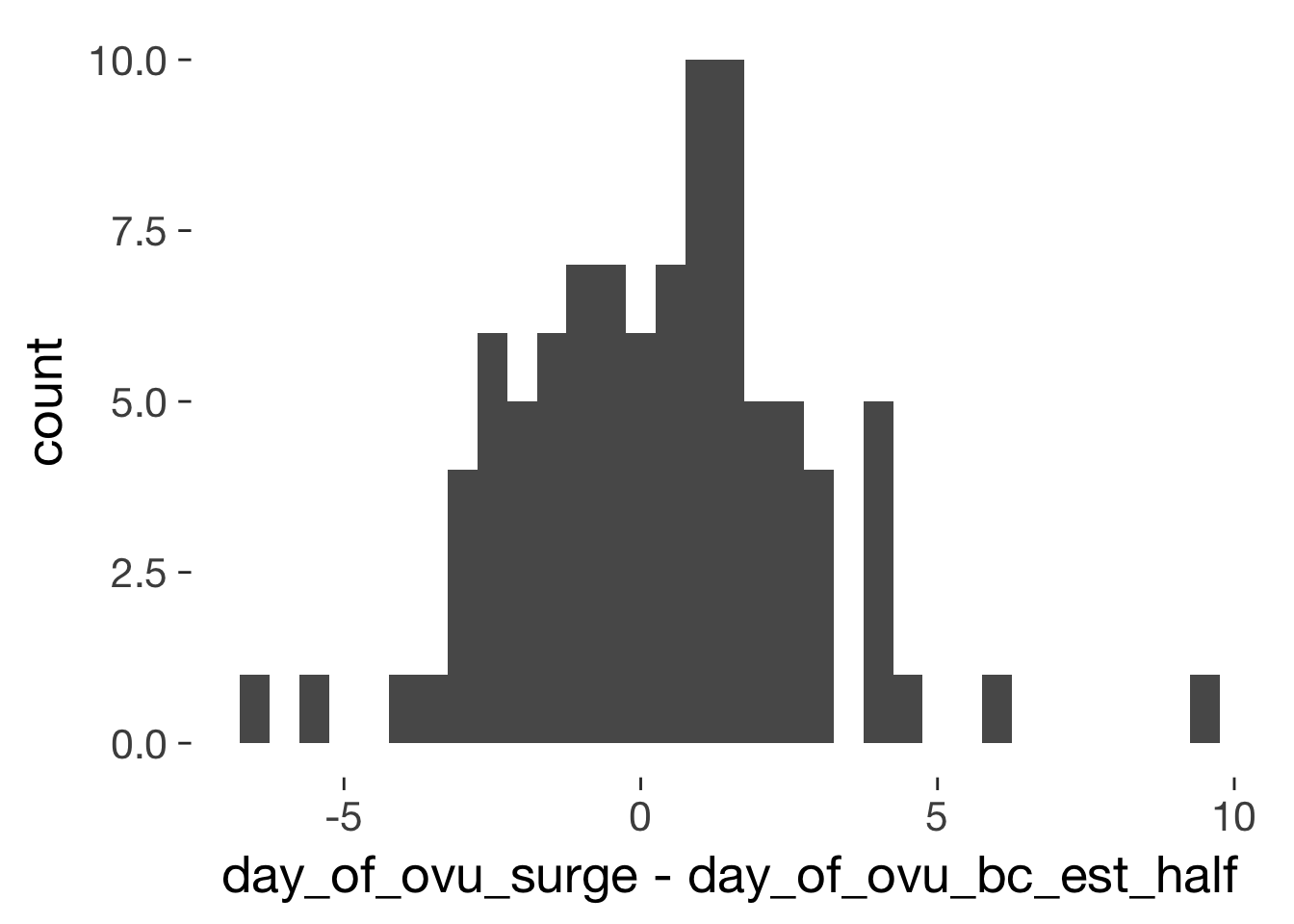## long dataset overview

library(DT)
datatable(long %>% select(-Age, -RelStat), rownames = F, filter = "top")